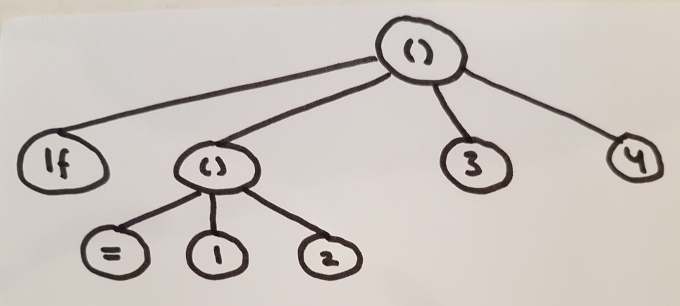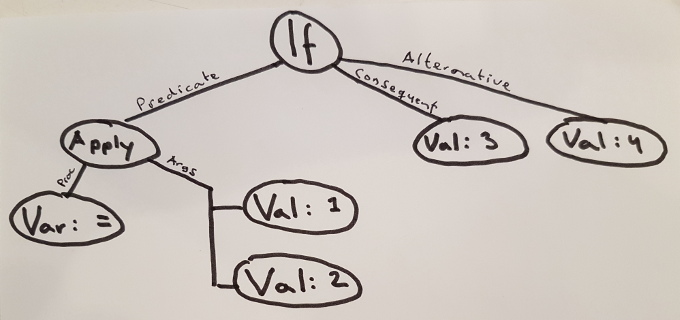To understand what we mean by form-analysis, and why it could be useful, consider again the model for evaluation presented in the previous article, which provides a definition of evaluation of s-expressions in environments.

A first step in this evaluation is a case-analysis on the form of the s-expression. For each evaluated s-expression, we first check whether the s-expression is an if-statement, lambda, function application or any of the other forms; only then we proceed accordingly. This case-analysis is reproduced below for reference, leaving out the semantics of evaluation for reasons of brevity.

• an atom which is either a string or a numbers is a value
• any other atom is a variable
• `(quote «s-expr»)` is a quoted expression
• `(quote «predicate» «consequent» «alternative»)` is an if-expression
• `(define «variable-name» «definition»)` is a definition
• `(lambda «parameter names» «body ...»)` is a lambda
• `(begin «... ...»)` is a sequence
• `(«procedure» «arguments ...»)` is a procedure application

In the process of evaluation of s-expressions, any given s-expression may be evaluated multiple times. Consider for example how, in our running example of the `factorial` procedure, when called with `(factorial 5)`, the body of that procedure is subjected to the case analysis for each invocation, for a total of 5 times. An interpreter that implements the above definition of evaluation directly, i.e. by operating on s-expressions, will repeat the case-analysis for each such evaluation.

### Static nature of the problem

However, the outcome of the analysis depends exclusively on certain static, structural aspects of the s-expression under consideration: first, whether it is an atom or a list; second, in case it’s a list, whether its first element is one of a specific set of atoms (`lambda`, `if`, …).

This means that, for any given s-expression, the result of the case-analysis will always be the same, independent of any dynamic aspects of the evaluation. Another way to say this is: the case-analysis can be done statically.1 That is, given an s-expression, we can identify which form it has, and recursively which forms that form is composed of. The output of such an analysis can be used as the input for a mechanism of evaluation.

### Why do static analysis?

Doing this is useful for a number of reasons:

First, as implied in the above, an interpreter which statically analyses its s-expression only once, before evaluation, can avoid repeating the same case analysis over an over at evaluation-time. This is beneficial for the interpreter’s performance.

Second, not every form is a legal form. For example, an s-expression denoting an if-form must have precisely 4 elements:  the symbol `if`,  a predicate,  a consequent and  an alternative; any other number of elements is illegal. A static analysis of forms will bring any malformed forms to light before evaluation-time. This means that such errors can be corrected sooner and with lower cost.

Third, a static model of the program’s forms is a prerequisite for nearly all other forms of static analysis. We better do it before proceeding.

A final argument for a separate phase of static form analysis can be found in the understanding of the material: doing form-analysis statically is trivial enough to be a good introduction into the subject of static analysis, but still non-trivial enough to provide some insights that can be useful when considering later analyses.

### Static analysis: a sketch

In the below we provide a rough sketch of a static form analysis.

Static form analysis is defined recursively on s-expressions. For each s-expression under consideration we do a case-analysis on the structure of the s-expression. The patterns on which we match correspond precisely to those enumerated above, i.e. we distinguish between various kinds of atoms, and for list-expressions we make a distinction based on their first element (`lambda`, `if`, …).

For each matched pattern an object of a specific type, with attributes which are specific to the form at hand, is constructed. For example, for an if-form we construct an if-form object, which has the 3 attributes predicte, consequent and alternative, each themselves being a form object.2

To construct the sub-forms out of s-expressions, we recurse. For most cases, the paths of recursion are analogous to those for evaluation. Some examples:

• for an if-form we recursively analyze the predicate, consequent and alternative.
• for a sequence we recursively analyze all s-expressions that make up the sequence.

For two cases, however, the recursion follows a different path than that of evaluation.

• For lambda-form we recursively analyze the body of the form – contrast this with the case of evaluation, in which the body of a lambda is not evaluated when the lambda is evaluated but instead stored in a procedure object.
• Analysis of function-application recursively analyses the application’s sub-expressions – contrast this with the case of evaluation, which proceeds to evaluate the body of the procedure object.

These two differences from the recursive definition of evaluation have the important consequence that the analysis is linear in time with the size of the s-expression under consideration including all its descendants – contrast this with evaluation, which may take infinite time.

Finally, a word on the well-formedness of forms: for forms which are not well-formed, such as a list-expression representing an if-form with any other number than 4 elements, a special malformed-form is constructed. For malformed forms we do not recurse.

### Context-dependent analysis

Nota bene: the fact that the analysis is recursively defined does not imply that an analysis of a given list-expression always recurses into all elements of that list. Instead, the paths of recursion are dependent on the form currently under consideration. In particular, the following sub-expressions are not subjected to further form-analysis at all:

• the form-identifying atoms (`if`, `lambda`, `begin` etc.) – these atoms determine the form associated with the s-expression containing them, but are not themselves subjected to further analysis.
• the quoted s-expressions of quote forms – the point of quoting is precisely that the quoted s-expression is not to be seen as a form: it is stored as-is as part of the quoted form.
• the single variable name in `define` – this variable name is stored as-is as an attribute of the definition.
• the list of parameter-names in `lambda` – stored as-is as a list of names.

This implies that we must approach the analysis in a top-down manner, because even the question as to whether we must analyze an s-expression to begin with is entirely dependent on its location in the tree.

### As a picture

Readers who prefer pictures over words may find the following section useful. In it, we show two diagrams.

In the first diagram an s-expression is presented, representing the expression `(if (= 1 2) 3 4)`.

In this diagram list-expressions simply show up as pairs of brackets, i.e. `()`, with the elements of those expressions below them.The second diagram represents the output of the form-analysis. In it, each special form has been recognized and labeled as such. The outgoing branches of the tree also have labels, which denote the specific role of each child-node in the tree.

Note that there is no 1-to-1 correspondence of nodes amongst the two trees. For example: the top-level s-expression `()` and its first element, the atom `if`, are combined into a single node in the tree representing form-analysis. Similarly: both the atoms `1` and `2` are folded into a single attribute of the function application representing all the function’s arguments.### Terminology

Finally, a few words on the chosen terminology.

Sussman & Abelson call the phase described here syntactic analysis. We have not followed that tradition, mainly because it is not obvious what noun to use to refer to the analyzed objects in that case.

Although the present phase has some properties that a reminiscent of “parsing”, we have refrained from using that word here, because it is generally associated with the parsing of strings – a phase which is entirely absent in the present project, given the fact that our point of departure is already a structured editor of s-expressions.

“Abstract Syntax Tree” was also ruled out as a term, because it does not tell us whether it’s the abstract syntax of s-expressions (the input of the current step) or the abstract syntax of forms (the output of the current step).

Regarding the term “forms” the following: In Lisp Forms are “any Lisp object that is intended to be evaluated”. Here we additionally mean “containing the relevant information on how this evaluation may take place given the object’s position in the full program tree”. Some people mean “Lisp Form” to be an implication of static correctness of the form. We don’t do that, and instead say that incorrect forms are a subset of forms. They are simply those lisp objects that are intended to be evaluated, but malformed.

### Concluding remarks

In this article, we have seen why static form analysis could be useful and how it could be implemented.

So far, we have only talked about the form-analysis of any given s-expression.

What we can want next is to implement an analysis that does a minimal amount of re-analyzing, given a previous version of the program, a completed analysis and a small change to the program.

Such an incremental form analysis is the subject of the next (as of yet to be written) article.

1. This is not a unique idea; it is, for example, explored in quite some detail in the Structure and Interpretation of Computer Programs in chapter 4.1.7, “Separating Syntactic Analysis from Execution”, p. 328.

2. How such an object is implemented depends on the language of implementation, e.g. in strongly typed functional languages one may implement such objects using algebraic data types, in Lisps using closures etc.